Example Questions

2 Next →

Example Question #11 : Proportion / Ratio / Rate

16 ounces of lemonade mix makes 2 gallons of lemonade (one gallon is equivalent to 4 quarts).

Quantity A: Amount of mix needed to make 3 quarts of lemonade

Quantity B: 6 ounces of mix

The relationship cannot be determined from the information given

Quantity A is greater

Quantity B is greater

The two quantities are equal

The two quantities are equal

Explanation:

2 gallons of lemonade equals 8 quarts of lemonade. To make 3 quarts of lemonade, you need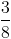of the amount needed to make 2 gallons of lemonade, or 6 ounces of mix.

Example Question #12 : How To Find Proportion

In a class withstudents, ifare taking Calculus andare taking Chinese, what is the lowest amount of students possible in the class that are taking both Calculus and Chinese?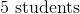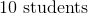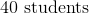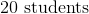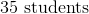Explanation:

This problem states that there arestudents in the class taking Calculus. Because the class has a total ofstudents, that means there are onlystudents in the class not taking Calculus. The problem also states that there arestudents in the class taking Chinese. Because onlystudents in the class aren't taking Calculus, this means that there is a minimum ofstudents in the class that are taking both Calculus and Chinese.

Example Question #13 : How To Find Proportion

If an a train is traveling at 8 feet per second, how many feet does it travel in 2 hours?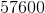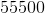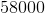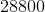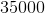Explanation:

For this problem, you must do conversions of from hours to seconds.

Two hours is equal 120 minutes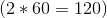.

120 minutes is equal to 7200 second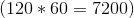.

The train travels 8 feet per second so in 7200 seconds it travels 57600 feet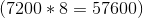.

2 Next →

Tired of practice problems?

Try live online GRE prep today.